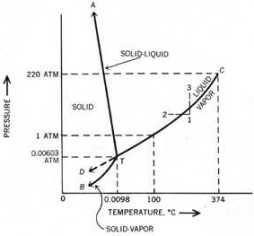Posted on

# Triple PointTriple point is the intersection on a phase diagram where three phases coexist in equilibrium. The most important application of triple point is water, where the three-phase equilibrium point consists of ice, liquid, and vapor. Before discussing triple point further, a basic understanding of the lines from Figure 1, the phase diagram of water, are first considered.

Take the line TC which gives the vapor pressure of liquid water up to the critical point C. Along this line, liquid and vapor coexist in equilibrium. At temperatures higher than that of point C, condensation does not occur at any pressure.

The line TA represents the vapor pressure of solid ice, which is a plot of the temperatures and pressures at which the solid and vapor are in equilibrium. Finally, line TB gives the melting point of ice and liquid water. The plot shows the temperatures and pressures at which ice and liquid water are in equilibrium.

(Note: At the dashed line TD, liquid water can be cooled below the freezing point to give supercooled water.)

The preceding paragraphs show that two phases are in equilibrium along the three solid lines. But when these lines intersect at one point C, three phases coexist in equilibrium. This intersection is the triple point, where a substance may simultaneously melt, evaporate, and sublime.